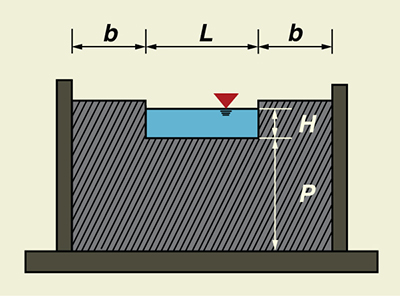Online Standard Contracted Rectangular Weir Calculator

131108

The program online_standard_contracted_rectangular calculates the discharge through a standard contracted rectangular weir. To be fully contracted, the overflow plate sides and ends must be located at a distance of at least 2H from the approach flow boundaries. The head is measured at a distance upstream of at least 4H from the weir. The computation procedure follows Section 9 of Chapter 7 of the USBR Water Measurement Manual.Fig. 1  Definition sketch for a standard contracted rectangular weir.

The formula for the standard contracted rectangular weir is the Francis equation. In U.S. Customary units, this equation is:

 Q = 3.33 (L - 0.2H) H3/2 

in which Q = discharge, in cfs, L = length of the weir crest, in ft, and H = head on the weir crest, in ft.

The accuracy of measurements obtained by Eq. 1 is considerably less than that obtainable with V-notch weirs. The accuracy of the discharge coefficient is ± 5 percent.

Equation 1 has a constant discharge coefficient (3.33), which facilitates computations. However, the coefficient does not remain constant for a ratio of head-to-crest H/L > 1/3, and the actual discharge exceeds that given by the equation. Francis' experiments were made on comparatively long weirs, most of them with crest length L = 10 ft and heads in the range 0.4 ft ≤ H ≤ 1.6 ft. Thus, the equation applies particularly to such conditions. USBR experiments on 6-in, 1-ft, and 2-ft weirs has shown that the Francis equation also applies fairly well to shorter crest lengths L, provided H/L ≤ 1/3.

Equation 1 is subject to the following restrictions:

1. Height-to-head ratio P/H ≥ 2.

2. Ratio b/H ≥ 2.

3. Length-to-head ratio:  L/H ≥ 3.

 131103